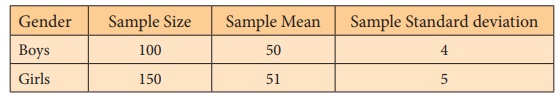Home | | Statistics 12th Std | Test of Hypotheses For Equality of Means of Two Populations (Population Variances are Unknown)

# Test of Hypotheses For Equality of Means of Two Populations (Population Variances are Unknown)

General Procedure for Test of Hypotheses For Equality of Means of Two Populations (Population Variances are Unknown): Procedure Steps, Example Solved Problems

TEST OF HYPOTHESES FOR EQUALITY OF MEANS OF TWO POPULATIONS (POPULATION VARIANCES ARE UNKNOWN)

### Procedure:

Step-1 : Let µX and σX2 be respectively the mean and the variance of Population -1. Also, let µY and σY2 be respectively the mean and the variance of Population -2 under study. Here σX2 and σY2 are assumed to be unknown.

Frame the null hypothesis as H0: µX = µY and choose the suitable alternative hypothesis from

(i) H1: µX ≠ µY (ii) H1: µX> µY (iii) H1: µX< µY

Step 2 : Let (X1, X2, …, Xm) be a random sample of m observations drawn from Population-1 and (Y1, Y2, …, Yn) be a random sample of n observations drawn from Population-2, where m and n are large (m ≥30 and n ≥30). Here, these two samples are assumed to be independent.

Step 3 : Specify the level of significance, α.

Step 4 : Consider the test statistici.e., the above test statistic is obtained from Z considered in the test described in Section 1.11 by substituting SX2 and SY2 respectively for σX2 and σY2

The approximate sampling distribution of the test statisticunder H0 is the N(0,1) distribution.

Step 5 : Calculate the value of Z for the given samples (x1, x2, ...,xm) and (y1, y2, …, yn) asHereandare respectively the values ofandfor the given samples.

Also, sx2 and sy2 are respectively the values of SX2 and SY2 for the given samples.

Step 6 : Find the critical value, ze, corresponding to α and H1 from the following tableStep 7 : Make decision on H0 choosing the suitable rejection rule from the following table corresponding to H1.### Example 1.11

A Model Examination was conducted to XII Standard students in the subject of Statistics. A District Educational Officer wanted to analyze the Gender-wise performance of the students using the marks secured by randomly selected boys and girls. Sample measures were calculated and the details are presented below:Test, at 5% level of significance, whether performance of the students differ significantly with respect to their gender.

### Solution:

Step 1 : Let μX and μY denote respectively the average marks secured by boys and girls in the Model Examination conducted to the XII Standard students in the subject of Statistics. Then, the null and the alternative hypotheses are

Null hypothesis: H0: µ X = µY

i.e., there is no significant difference in the performance of the students with respect to their gender.

Alternative hypothesis: H1 : µ X µY

i.e., performance of the students differ significantly with the respect to the gender. It is a two-sided alternative hypothesis.

Step 2 : Data

The given sample information areSince m ≥ 30 and n ≥ 30, both the samples are large.

Step 3 : Level of significance

α= 5%

Step 4 : Test statistic

The test statistic under H0 isThe sampling distribution of Z under H0 is the N(0,1) distribution.

Step 5 : Calculation of the Test Statistic

The value of Z is calculated for the given sample informations fromStep 6 : Critical value

Since H1 is a two-sided alternative, the critical value at 5% level of significance is ze = z0.025 = 1.96.

Step 7 : Decision

Since H1 is a two-sided alternative, elements of the critical region are determined by the rejection rule |z0| ≥ z0 . Thus it is a two-tailed test. But, |z0|= 1.75 is less than the critical value ze = 1.96. Hence, it may inferred as the given sample information does not provide sufficient evidence to reject H0. Therefore, it may be decided that there is no sufficient evidence in the given sample to conclude that performance of boys and girls in the Model Examination conducted in the subject of Statistics differ significantly.

Tags : Procedure Steps, Example Solved Problems | Statistics , 12th Statistics : Chapter 1 : Tests of Significance - Basic Concepts and Large Sample Tests
Study Material, Lecturing Notes, Assignment, Reference, Wiki description explanation, brief detail
12th Statistics : Chapter 1 : Tests of Significance - Basic Concepts and Large Sample Tests : Test of Hypotheses For Equality of Means of Two Populations (Population Variances are Unknown) | Procedure Steps, Example Solved Problems | Statistics